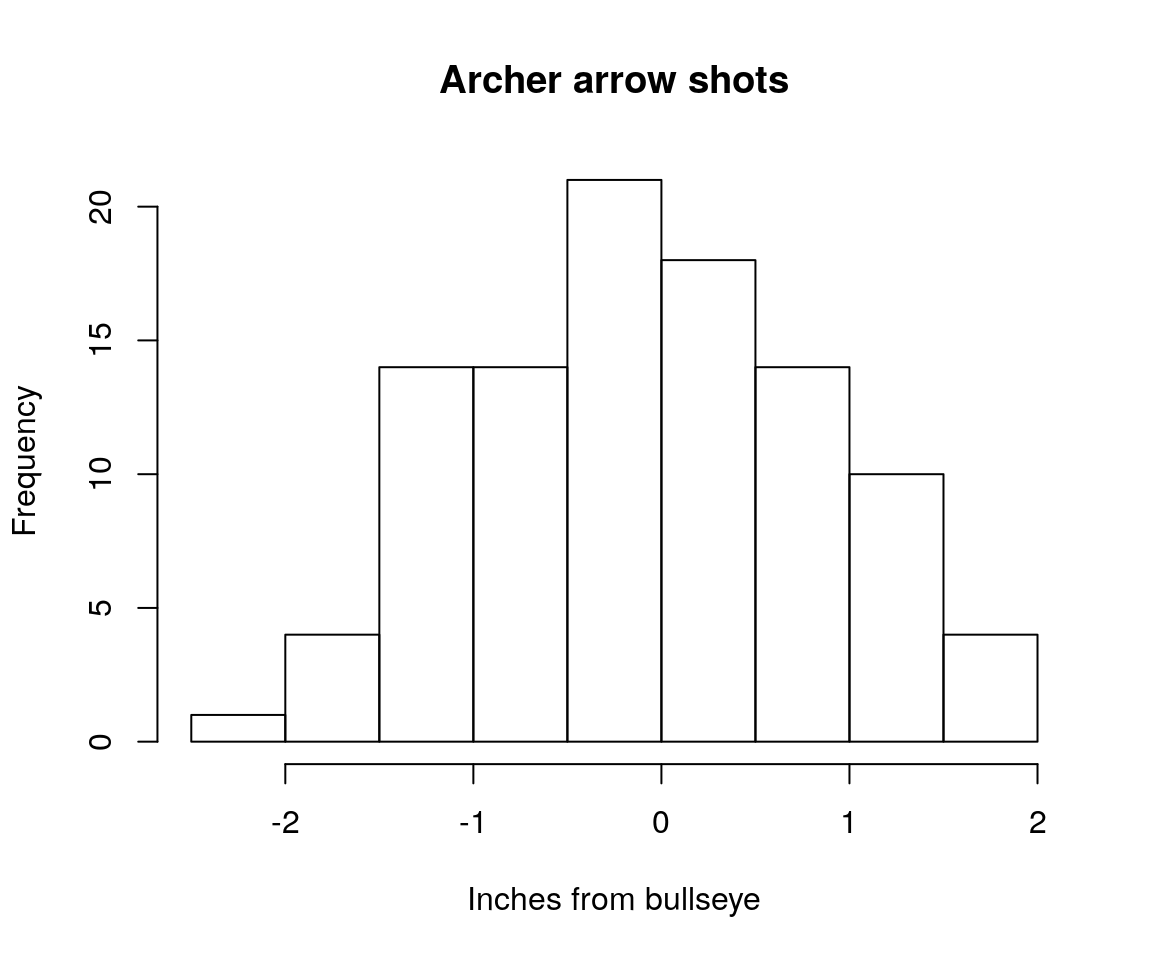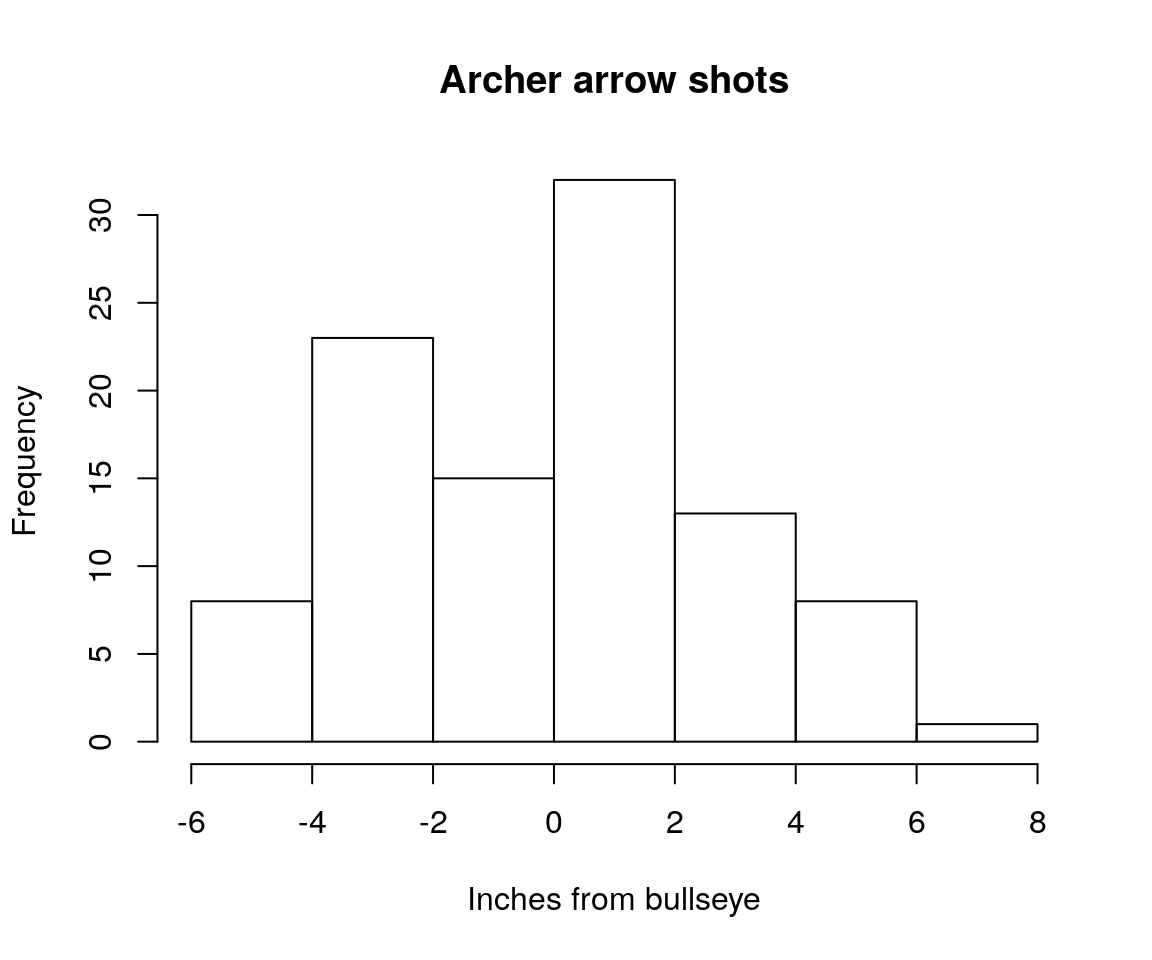# Research and Teaching With RevBayes

## Overview

• Introduction to Coding to Learn
• Introduction to graphical modeling
• How graphical modeling makes phylogenetic estimation more flexible
• Using RevBayes in the classroom

## Learning to Code

We’re all familiar with the idea of learning to code

## Learning to Code

We’re all familiar with the idea of learning to code

• Maybe students in your degree program take an upper-division course where codin is the aim

## Learning to Code

We’re all familiar with the idea of learning to code

• Maybe students in your degree program take an upper-division course where codin is the aim
• This is the “learn to code” framework. Being able to produce code is the end goal

## Learning to Code

• If we confine coding to niche classes, we’re likely to miss much of the population
• Large proportion of students who seek out computational training have seen computation in high school

## Learning to Code

• If we confine coding to niche classes, we’re likely to miss much of the population
• Large proportion of students who seek out computational training have seen computation in high school

== Confining learning to people who are already convinced of the utility

## Learning to Code

• If we confine coding to niche classes, we’re likely to miss much of the population
• Large proportion of students who seek out computational training have seen computation in high school

== Students get coding either late in undergrad, or after they’ve been mucking around unproductively with data

## Code to Learn

Coding doesn’t have to be the main focus of what you’re doing.

## Code to Learn

Coding doesn’t have to be the main focus of what you’re doing

• Code to learn: work with data computationally to develop biological insight

## Code to Learn

Coding doesn’t have to be the main focus of what you’re doing

• Code to learn: work with data computationally to develop biological insight
• Example: Rather than talking about Bergmann’s Rule, have students download occurrence data from GBIF and key it to a trait database to observe body size along longitudinal gradients

## Code to Learn

Citations for many of the things in the previous couple slides can be found in this manuscript

## Code to Learn

Coding doesn’t have to be the main focus of what you’re doing

• Systematics is a field ripe with opportunities
• To understand systematics, you need to know some
• Biology
• Statistics
• Math
• Geology & Earth history

## Code to Learn

Coding doesn’t have to be the main focus of what you’re doing

• Systematics is a field ripe with opportunities
• In performing systematics research, we learn about
• Biology
• Statistics
• Math
• Geology & Earth history

## RevBayes

RevBayes is a Bayesian modeling software underlain by a graphical model framework

## RevBayes

RevBayes is a Bayesian modeling software underlain by a graphical model framework

• RevBayes implements an R-like statistical modeling language called Rev

## RevBayes

RevBayes is a Bayesian modeling software underlain by a graphical model framework

• RevBayes implements an R-like statistical modeling language called Rev
• The design of the language is meant to give users control over the details of their analysis

## The Graphical Modeling Framework

• What is a model?

## The Graphical Modeling Framework

• What is a model?
• A model uses mathematics to describe a process, or the behavior of a system
• Important facets of the generating process of the data are written down as parameters

## The Graphical Modeling Framework

• What is a model?
• A model uses mathematics to describe a process, or the behavior of a system
• Important facets of the generating process of the data are written down as parameters
• A graphical model represents the relationships and dependencies between model paramters

## A simple case

• An archer: Let’s say the archer is a pretty good archer

## A simple case

good_shots <- rnorm(n= 100, mean= 0, sd = 1)
hist(good_shots, main="Archer arrow shots",  xlab = "Inches from bullseye")## A simple case

• An archer: Let’s say the archer is a pretty bad archer

## A simple case

bad_shots <- rnorm(n= 100, mean= 0, sd = 5)
hist(bad_shots, main="Archer arrow shots",  xlab = "Inches from bullseye")## A not so simple case

• It’s easy to describe a model where we are manipulating one parameter
• But we rarely have such a simple case when we’re analysing biological data
• We’re describing the mechanisms that generated our observed data
• In the case of a tree, this is the process of molecular or morphological evolution

## A not so simple case

• This is where graphical models come in

## A not so simple case

• This is where graphical models come in
• A graphical model represents the joint probability distribution as a graph

## A not so simple case

• This is where graphical models come in
• A graphical model represents the joint probability distribution as a graph
• Nodes are variables. Edges represent dependencies between nodes.

## A not so simple case

• This is where graphical models come in
• A graphical model represents the joint probability distribution as a graph
• Nodes are variables. Edges represent dependencies between nodes.

## A not so simple case

• This is where graphical models come in
• A graphical model represents the joint probability distribution as a graph
• Nodes are variables. Edges represent dependencies between nodes.

## A not so simple case

• This is where graphical models come in
• A graphical model represents the joint probability distribution as a graph
• Nodes are variables. Edges represent dependencies between nodes.

Pr( data | model )

## Phylogenetical Complexity

• This is where graphical models come in
• A graphical model represents the joint probability distribution as a graph
• Nodes are variables. Edges represent dependencies between nodes.

## Who cares about graphical modeling?

• In previous iterations of software, you might have to email a developer and ask them to implement something
• RevBayes’ graphical model framework emphasizes flexibility

## Who cares about graphical modeling?

Over the next two hours, we’re going to make the case that this framework enables flexible, code-to-learn classrooms